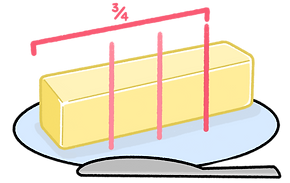top of page## Fractions

Fractions are used to represent parts of a whole thing. Many examples of fractions are shown using circular objects,  however other shapes can also be split into fractions like squares, rectangles, triangles.

The top number of a fraction shows how many parts of a whole that you have, and is called the numerator.

The bottom of a fraction shows the total numbers of elements that make up the whole, and is called the denominator.#### A whole orange can be cut into 2 halves (½). Then that half can be cut in half again, leaving 4 quarter (¼) slices.## Proper Fractions

Proper fractions are used to measure the individual parts of one whole thing. In cases like this, the numerator is smaller or equal to the denominator:

½ ,  ¼ ,  ⅔ , ¾ ,  ⁴⁄₁₆

## ​

Improper fractions are used when the total number of parts make up more than one whole. For example, if you have 5 quarter slices of an orange, this is represented by the fraction ⁵⁄₄ .

## Like Fractions

Fractions with the same denominators are called Like Fractions, and can be easily added together by adding the numerators and leaving the denominator the same.

⅓ + ⅔ = ³⁄₃ = 1

²⁄₁₀ + ⁵⁄₁₀ = ⁷⁄₁₀

bottom of page